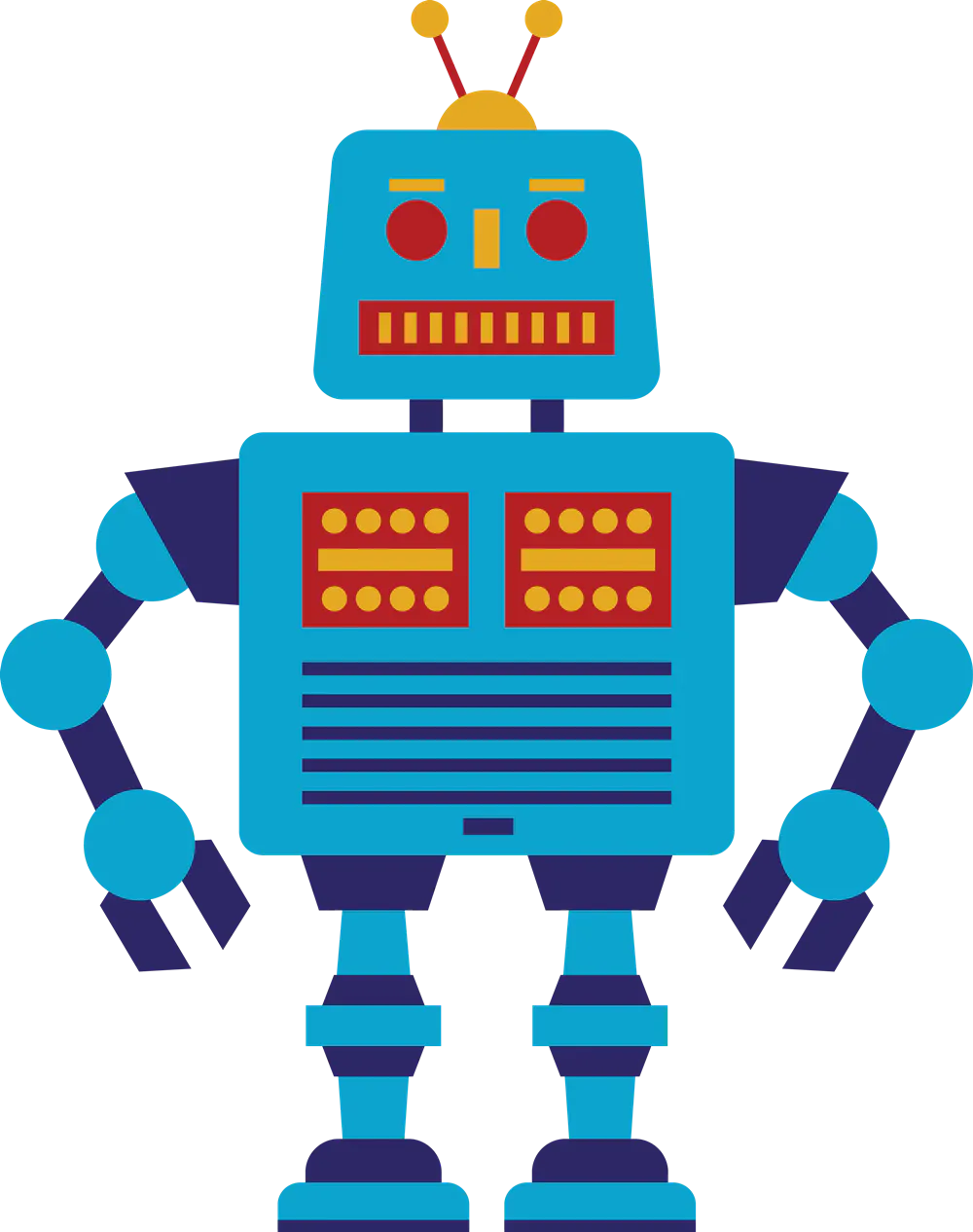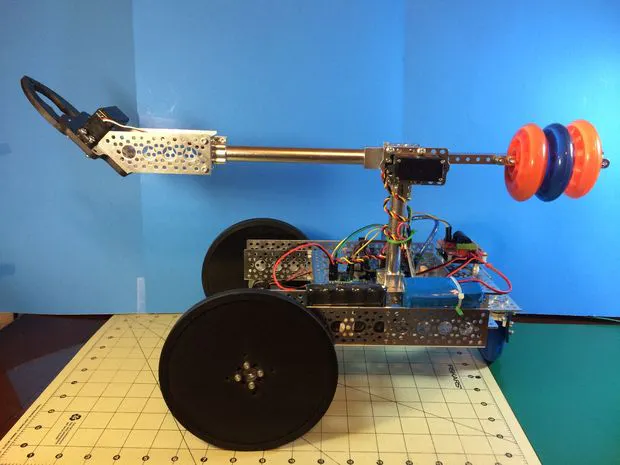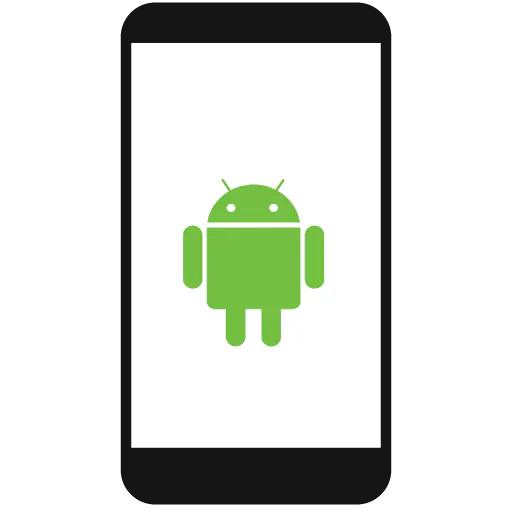# Boom and Gripper bot

Remote controlled Boom and gripper bot. Control via Phone or a Python GUI.

IntermediateWork in progress1,084## Things used in this project

### Hardware componentsAndroid device
×1

## Code

### boombot_roboclaw.ino

C/C++
```/*
Bluetooth Boom-Gripper bot.

Goal in life...
Follows your commands sent magically through the air! Or from USB :)

Written by Scott Beasley - 2015
Free to use or modify. Enjoy.
*/

#include

// Various time delays used for driving and servo
#define TURNDELAY 475
#define TURNDELAY45 235

// Pololu servo controller commands
#define SETSPEED 0x01
#define SETPOS 0x04

/*
Globals area.
*/

// Create the motor, servo objects to interface with
SoftwareSerial Serial_servo (10, 11);
SoftwareSerial Serial_motors (10, 12);

void setup ( )
{
// Change the baud rates here if different than below
Serial.begin (9600); // Bluethooth connection
Serial_servo.begin (9600);
Serial_motors.begin (19200);

halt ( );
set_servo_speed (0, 10); // Set the servo movement speed
set_servo_speed (1, 10); // to make them move a bit smoother
home_boom ( );
home_gripper ( );

delay (500);
}

void loop ( )
{
byte command = 0, val = 0;
// The following need to stay intact during the life of the
// session so they are made 'static'. This keeps us from
// having to have them as globals
static byte speed = 1; // slow speed
static byte speed_offset = 40;
static byte turn_style = 1; // and with hard turns
static byte boom_val = 60;
static byte gripper_val = 60;

if (Serial.available ( ) > 0) {
// read the incoming command byte
}

switch (command) {
case 'w':
go_forward (speed_offset);
//Serial.println ("Going Forward");

if (dist == 1) {
delay (750);
halt ( );
} else if (dist == 2) {
delay (1250);
halt ( );
}

break;

case 'z':
go_backward (speed_offset);
//Serial.println ("Going Backwards");

if (dist == 1) {
delay (750);
halt ( );
} else if (dist == 2) {
delay (1250);
halt ( );
}

break;

case 'a':
go_left (speed_offset, turn_style);
delay (TURNDELAY);
halt ( );
//Serial.println ("Turning Left");
break;

case 's':
go_right (speed_offset, turn_style);
delay (TURNDELAY);
halt ( );
//Serial.println ("Turning Right");
break;

case 'q':
go_left (speed_offset, turn_style);
delay (TURNDELAY45);
halt ( );
//Serial.println ("Turning Left");
break;

case 'e':
go_right (speed_offset, turn_style);
delay (TURNDELAY45);
halt ( );
//Serial.println ("Turning Right");
break;

case 'h':
halt ( );
//Serial.println ("Halting");
break;

case '>':
// Gripper X move sends servo set value
gripper_val = val;

// We limit the value to real movemovent limits of the setup
set_gripper (val);
//Serial.println ("GripperX");
break;

case '^':
// Gripper Y move sends servo set value
boom_val = val;

// We limit the value to real movemovent limits of the setup
set_boom (val);
//Serial.println ("GripperY");
break;

case 'c':
gripper_val = 60;
boom_val = 60;
home_boom ( );
home_gripper ( );

//Serial.println ("GripperHome");
break;

case 'v':
// Set the spped the bot will move; 1 = slowest, 3 = fastest

if (speed == 1) {
speed_offset = 40;    // Slow
} else if (speed == 2) {
speed_offset = 20;    // Med
} else if (speed == 3) {
speed_offset = 0;     // Fast!
}

//Serial.println ("Set speed");
break;

case 'd':
// Set how long the bot will move; 1 = short, 2 medium,
// 3 = continuous
//Serial.println ("Move distance set");
break;

case 't':
// Set the way the bot does turns, one wheel (soft) or two (hard)

//Serial.println ("Turn style");
break;

case '+': // Speed set from phone
speed++;
if (speed >= 4)
speed = 1;

if (speed == 1) {
speed_offset = 40;    // Slow
} else if (speed == 2) {
speed_offset = 20;    // Med
} else if (speed == 3) {
speed_offset = 0;     // Fast!
}

break;

case '-': // Dist moved from phone
dist++;

if (dist >= 4)
dist = 1;
//Serial.println ("Move distance set");
break;

case ',': // Open Gripper from phone
gripper_val += 10;

if (gripper_val >= 179)
gripper_val = 179;

set_gripper (gripper_val);

//Serial.println ("Gripper set");
break;

case '.': // Close Gripper from phone
gripper_val -= 10;

if (gripper_val <= 0)
gripper_val = 0;

set_gripper (gripper_val);

//Serial.println ("Gripper set");
break;

case '0': // Lower boom from phone
boom_val += 10;

if (boom_val >= 179)
boom_val = 179;

set_boom (boom_val);

//Serial.println ("Boom set");
break;

case '1': // Raise boom from phone
boom_val -= 10;

if (boom_val <= 0)
boom_val = 0;

set_boom (boom_val);

//Serial.println ("Boom set");
break;

case 255: // Sent after all gripper commands
Serial.flush ( );
break;
}

Serial.flush ( );
delay(125);
}

void go_forward (byte speed_offset)
{
//Serial.println ("Going forward...");
Serial_motors.write ((byte)1 + speed_offset);
Serial_motors.write ((byte)128 + speed_offset);
}

void go_backward (byte speed_offset)
{
//Serial.println ("Going backward...");
Serial_motors.write ((byte)127 - speed_offset);
Serial_motors.write ((byte)255 - speed_offset);
}

void go_left (byte speed_offset, byte turn_style)
{
//Serial.println ("Going left...");
if (turn_style == 1)
Serial_motors.write ((byte)1 + speed_offset);
else
Serial_motors.write ((byte)64); // Turn off the left motor (soft turn)

Serial_motors.write ((byte)255 - speed_offset);
}

void go_right (byte speed_offset, byte turn_style)
{
//Serial.println ("Going right...");

Serial_motors.write ((byte)127 - speed_offset);

if (turn_style == 1)
Serial_motors.write ((byte)128 + speed_offset);
else
Serial_motors.write ((byte)192); // Turn off the right motor (soft turn)
}

void halt ( )
{
//Serial.println ("Halt!");
Serial_motors.write ((byte)0);
}

// Set a servos position
//   servo_id is the servo number (0-7)
//   angle is the abs position from 500 to 5500
void move_servo (int servo_id, int angle)
{
unsigned char buff = {0x80, 0x01, SETPOS, 0x00, 0x00, 0x00};

unsigned int temp;
unsigned char data_hi, data_low;

temp = angle & 0x1f80;
data_hi = temp >> 7;
data_low = angle & 0x7f;

buff = servo_id; //servo number
buff = data_hi; //data1
buff = data_low; //data2

Serial_servo.write (buff, 6);
Serial_servo.flush ( );
}

void set_servo_speed (int servo_id, int speed)
{
unsigned char buff = {0x80, 0x01, SETSPEED, 0x00, 0x00};
if (speed > 127)
speed = 127;

buff = lowByte (servo_id);
buff = lowByte (speed);
Serial_servo.write (buff, 5);
Serial_servo.flush ( );
}

void set_boom (byte angle)
{
move_servo (0, map (angle, 0, 179, 2500, 4000));
}

void set_gripper (byte angle)
{
move_servo (1, map (angle, 0, 179, 2500, 4000));
}

void home_boom ( )
{
move_servo (0, 3000);
}

void home_gripper ( )
{
move_servo (1, 3000);
}
```

### rover.py

Python
```#!/usr/bin/env python
#
# For Linux, BSD or Mac OSX you can chmod +x on this script to make executable
#
###########
# Rover control app
#
# Allows for simple multi-byte commands.
#
# Written by Scott Beasley - 2015
# Free to use or modify. Enjoy.
#
###########

import sys
import serial
import time
from Tkinter import *
import tkFont
import tkMessageBox

# Create the window for the application
class App (Frame):
# Make the window
def createWidgets (self):
self.connected = False
self.message = StringVar ( )

# Make a little font for the gripper set buttons.
helv6 = tkFont.Font (family = 'Helvetica',
size = 6, weight = 'normal')

self.frame = Frame (self.master)
self.frame.pack ( )

self.f1 = Frame (self.frame)
self.l1 = Label (self.f1, text = "Comm Port: ")
self.l1.pack (side = LEFT)
self.comm_entry = Entry (self.f1, bd = 5, name = "comm_entry")
self.comm_entry.pack (side = LEFT)
self.connectButton = Button (self.f1, text = "Connect",
command = self.SerialConnect,
name = "b_connect")
self.connectButton.pack (side = LEFT)
self.disconnectButton = Button (self.f1, text = "Disconnect",
command = self.SerialDisconnect,
name = "b_disconnect")
self.disconnectButton.pack (side = RIGHT)
self.f1.grid (row = 0, column = 0)

self.f2 = LabelFrame (self.frame, bd = 3, relief = "groove",
text="Ground Control")

self.g_vert_fm = Frame (self.f2)
self.grip_vert = Scale (self.g_vert_fm, from_ = 0, to = 180)
# Bind on mouse button 1 release to set the boom position
self.grip_vert.bind ('', self.GripperY)
self.grip_vert.grid (row = 0, column = 0, rowspan = 4, sticky = W)
self.master.bind ("", self.GripperY)
self.g_vert_fm.grid (row = 0, column = 0, rowspan = 4, sticky = W)

self.leftforwardButton = Button (self.f2, text = "\\",
command = self.TurnLeft45,
name = "b_left_forward")
self.leftforwardButton.grid (row = 0, column = 1)
self.master.bind ("<a rel="nofollow">", self.TurnLeft45)

self.leftButton = Button (self.f2, text = "<",
command = self.TurnLeft, name = "b_left")
self.leftButton.grid (row = 1, column = 1)
self.master.bind ("</a><a rel="nofollow">", self.TurnLeft)

self.rightforwardButton = Button (self.f2, text = "/",
command = self.TurnRight45,
name = "b_right_forward")
self.rightforwardButton.grid (row = 0, column = 3)
self.master.bind ("</a><a rel="nofollow">", self.TurnRight45)

self.haltButton = Button (self.f2, text = "Halt!",
command = self.Halt, name = "b_halt")
self.haltButton.grid (row = 1, column = 2)
self.master.bind ("</a><a rel="nofollow">", self.Halt)

self.rightButton = Button (self.f2, text=">",
command = self.TurnRight, name = "b_right")
self.rightButton.grid(row = 1, column = 3)
self.master.bind ("</a><a rel="nofollow">", self.TurnRight)

self.upButton = Button (self.f2, text="^",
command = self.Forward, name = "b_forward")
self.upButton.grid (row = 0, column = 2)
self.master.bind ("</a><a rel="nofollow">", self.Forward)

self.leftdownButton = Button (self.f2, text = "/",
command = self.TurnRight45,
name = "b_left_down")
self.leftdownButton.grid (row = 2, column = 1)

self.downButton = Button (self.f2, text="V",
command = self.Reverse, name = "b_reverse")
self.downButton.grid (row = 2, column = 2)
self.master.bind ("</a><a rel="nofollow">", self.Reverse)
self.f2.grid (row = 1, column = 0, pady = 25)

self.rightdownButton = Button (self.f2, text = "\\",
command = self.TurnLeft45,
name = "b_right_down")
self.rightdownButton.grid (row = 2, column = 3)

self.g_horz_fm = Frame (self.f2)
self.grip_horz = Scale (self.g_horz_fm, from_ = 0, to = 180,
orient = HORIZONTAL)
# Bind on mouse button 1 release to set the gripper position
self.grip_horz.bind ('</a><a rel="nofollow">', self.GripperX)
self.grip_horz.grid (row = 0, column = 0, columnspan = 7, sticky = E)

self.master.bind ("<u>", self.GripperX)
self.g_horz_fm.grid (row = 4, column = 0, columnspan = 7, sticky = E)
self.master.bind ("</u></a><a rel="nofollow"><u>", self.GripperHome)

self.f_spd_dist = Frame (self.frame)
self.l_speed = Label (self.f_spd_dist, text = "  Speed: ")
self.l_speed.grid (row = 0, column = 0)
self.speed_spin = Spinbox (self.f_spd_dist, values = (1, 2, 3),
width = 2, command = self.SetSpeed)
self.speed_spin.grid (row = 0, column = 1)
self.l_dist = Label (self.f_spd_dist, text = "  Distance: ")
self.l_dist.grid (row = 0, column = 3)
self.dist_spin = Spinbox (self.f_spd_dist,
values = ('Short', 'Medium', 'Continuous'),
width = 10, command = self.SetDistance)
self.dist_spin.grid (row = 0, column = 4)
self.l_turns = Label (self.f_spd_dist, text = "  Turns: ")
self.l_turns.grid (row = 0, column = 5)
self.turns_spin = Spinbox (self.f_spd_dist,
values = ('Hard', 'Soft'),
width = 5, command = self.SetTurns)
self.turns_spin.grid (row = 0, column = 6)
self.f_spd_dist.grid (row = 2, column = 0)

self.f3 = Frame (self.frame)
self.l2 = Label (self.f3, text = "Last action: ")
self.l2.pack (side = LEFT)
self.l3 = Label (self.f3, text=" ", textvariable = self.message)
self.l3.pack (side = RIGHT)
self.f3.grid (row = 5, column = 0, pady = 8)

# Set the state of the bot control buttons. Enable when connected,
# Disabled otherwise.
def CtrlButtonsState (self, bstate):
self.leftforwardButton.config (state = bstate)
self.leftButton.config (state = bstate)
self.rightforwardButton.config (state = bstate)
self.rightButton.config (state = bstate)
self.upButton.config (state = bstate)
self.leftdownButton.config (state = bstate)
self.downButton.config (state = bstate)
self.rightdownButton.config (state = bstate)
self.haltButton.config (state = bstate)
self.disconnectButton.config (state = bstate)
self.grip_horz.config (state = bstate)
self.grip_vert.config (state = bstate)

# Set the state of the comm port entry. Enable when not connected,
# Disabled when the bot is connected.
def ConnCtrlsState (self, bstate):
self.connectButton.config (state = bstate)
self.comm_entry.config (state = bstate)

# Connect to the comm port typed in the comm entry field.
def SerialConnect (self):
try:
# Change the baud rate here if diffrent then 9600
self.ser = serial.Serial (self.comm_entry.get ( ), 9600)
except IOError:
return

self.ConnCtrlsState (DISABLED)
self.CtrlButtonsState (NORMAL)
self.message.set ("SerialConnect")
self.connected = True
time.sleep (3) # Dwell a bit for the connection to happen

# Do some after connection bot talking.
if self.connected == True:
self.setbotParms ( )

# Disconnect from the bot (close the comm port).
def SerialDisconnect (self):
try:
# Send a Halt command just in case the bot is still moving.
self.send_cmd ('h', "Halt!")
time.sleep (1)
self.ser.close ( )
except IOError:
print "Could not close port..."

self.message.set ("SerialDisconnect")
self.ConnCtrlsState (NORMAL)
self.CtrlButtonsState (DISABLED)
self.connected = False
time.sleep (2) # Dwell a bit for the disconnection to happen

# Send the command to the open comm port
# the action input variable is a tuple that allows for multy part commands
def send_cmd (self, action, msg):
if self.connected == True:
for val in action:
self.ser.write (val)
self.ser.flush ( )
self.message.set (msg)

# Send the bot a turn-left command.
def TurnLeft (self, event = None):
self.send_cmd ('a', "Left")

# Send the bot a turn-left-up command.
def TurnLeft45 (self, event = None):
self.send_cmd ('q', "Left45")

# Send the bot a turn-right command.
def TurnRight (self, event = None):
self.send_cmd ('s', "Right")

# Send the bot a turn-right-up command.
def TurnRight45 (self, event = None):
self.send_cmd ('e', "Right45")

# Send the bot a Forward command.
def Forward (self, event = None):
self.send_cmd ('w', "Up")

# Send the bot a Reverse command.
def Reverse (self, event = None):
self.send_cmd ('z', "Down")

# Send the bot a Halt command.
def Halt (self, event = None):
self.send_cmd ('h', "Halt!")

# Set the gripper (X).
def GripperX (self, event = None):
# Read the slider control and send the value to the bot controller
# Note: 0 is all the way closed and 180 is all the way open
# This is a multi-byte command. The command + the servo set val
# + a buffer clear command (optional)
grp_change = ('>', chr (self.grip_horz.get ( )), chr (255))
self.send_cmd (grp_change, "Gripper X")
self.boomchange = None

# Set the gripper Y.
def GripperY (self, event = None):
# Read the slider control and send the value to the bot controller
# Note: 0 is all the way up and 180 is all the way down
# This is a multi-byte command. The command + the servo set val
# + a buffer clear command (optional)
grp_change = ('^', chr (self.grip_vert.get ( )), chr (255))
self.send_cmd (grp_change, "Gripper Y")
self.gripperchange = None

# Set the gripper to the "home" position.
def GripperHome (self, event = None):
self.send_cmd (('c', chr (255)), "Gripper Home")
self.grip_horz.set (90)
self.grip_vert.set (90)

# Set the speed of the rover.
def SetSpeed (self, event = None):
spd_str = self.speed_spin.get ( )

if spd_str == "1":
speed = 1
if spd_str == "2":
speed = 2
if spd_str == "3":
speed = 3

# Sends a multi-byte command to set the speed.
self.send_cmd (('v', chr (speed)), "Set Speed")

# Set the distance travled when a for or rev is issued to
# the rover.
def SetDistance (self, event = None):
dist = 1
dist_str = self.dist_spin.get ( )

if dist_str == "Short":
dist = 1
if dist_str == "Medium":
dist = 2
if dist_str == "Continuous":
dist = 3

# Sends a multi-byte command to set the distance.
self.send_cmd (('d', chr (dist)), "Set Distance")

# Set the turn style to use turning.
def SetTurns (self, event = None):
turn = 1
turn_str = self.turns_spin.get ( )

if turn_str == "Hard":
turn = 1
if turn_str == "Soft":
turn = 2

# Sends a multi-byte command to set the turn style.
self.send_cmd (('t', chr (turn)), "Set Turns")

def setbotParms (self):
self.Halt ( )
self.GripperHome ( )
self.SetSpeed ( )
self.SetDistance ( )
self.SetTurns ( )

def __init__ (self, master = None):
Frame.__init__ (self, master)
self.pack ( )
self.createWidgets ( )
self.CtrlButtonsState (DISABLED)

# Kick off the GUI (Tk) then size and title the app window
def main ( ):
root = Tk ( )
root.geometry ("450x360")
root.wm_title ("Rover Control Center (RCC)")
app = App (master = root)
app.mainloop ( )

if __name__ == '__main__':
main ( )
</u></a>
```

## Credits

### Scott Beasley

9 projects • 56 followers
Well odd